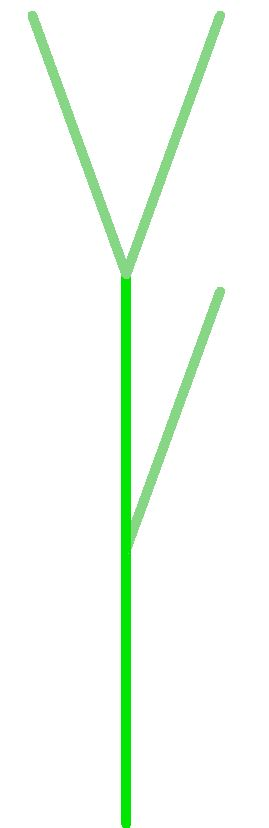MUN Computer Science 1002 - Lab 7 Recursive definitions

Review:

• Lectures 20-23.

Vocabulary

• Sum, product, factorial notation
• Sequences, their recursive definitions and closed forms.
• Arithmetic and geometric progressions. Fibonacci sequence. Partial sums.
• Recursive definitions of fractals, trees, formulas, arithmetic expressions.
• Context-free grammars, derivations, parse trees.

Individual work

Solve each of the following exercises.

1. Compute the following (you can use a calculator).
1. $\Sigma_{i=2}^5 i^2$,
2. $\Pi_{i=0}^3 (i+2)$
3. $(10-6)!$
2. List the first five elements in the following sequences.
1. Arithmetic sequence with c=10 and d = 4.
2. $(n!)/2^n$ for $n \in \mathbb{N}$. (Hint: define $0!=1$).
3. $F^2_n$, $n \in \mathbb{N}$, where $F_n$ is the nth Fibonacci number.
3. Suppose that expressions on binary numbers are defined as follows.
• Base: any binary number (that is, a sequence of 0s and 1s) is a an expression on binary numbers.
• Recursion: if a and b are two expressions on binary numbers, then so are (a) AND (b), (a) OR (b), (a) XOR (b).
According to this definition, which of the following are valid expressions on binary numbers?
1. (00100) AND ( (0011) OR (11))
2. 00011 XOR 11100
3. (00111)
4. Draw the base and first two iterations of the following fractals.
1. Base: a line segment (with a direction). Recursive step: replace each line segment with2. Base: a square. Recursive step: Make each (empty) square a 3x3 grid (mark off 1/3 and 2/3 of each side and draw vertical and horizontal lines), and shade the middle square in the grid.

Group exercises

For this part of the lab, you will be working in groups of three. In the first part of the exercise, each of three people in the group picks one of the topics below, and solves the corresponding questions. Then, each of you will explain to two of your peers how you have solved these questions. Your explanation should be good enough that they can then solve similar questions on their own.

Topic 1: Recursive definitions of sets.

Give a recursive definition of the following sets (directly, without using grammars).
• Set of all non-empty even-length strings over $\{a,b,c\}$.
• Set of all even natural numbers (your base case should still be finite).

Topic 2: Grammars as recursive definitions of sets

Recall that a (context-free) grammar consists of rules of the form $A \to w$, where $A$ is a variable, and $w$ is a string of variables and terminals (possibly empty). For each of the following languages, define a grammar that produces this language.

• A language of all strings over $\{0, 1\}$ which end with 1.
• A language of all strings that contain at least two 0s.

Topic 3: Parse trees and derivations

Let a language over $\{0,1\}$ be defined by the grammar $S \to S00 | 0S0 | 1 | \lambda$, where $\lambda$ is an empty string.

• Give two examples of strings in the language (other than ones in subquestion below), and two examples of strings not in this language.
• Draw parse trees to derive "000000" and "01000".

Review after the group activity

Consider the language of all palindromes over $\{a,b,c\}$ (that is, a set of all strings that read the same forwards and back).

1. Give two examples of strings in this language, and two examples not in this language.
2. Give a recursive definition of all palindromes over $\{a,b,c\}$. Hint: an empty string is a palindrome, and they can be even or odd length.
3. Give a grammar generating this language.
4. Draw parse trees for a string $aabcbaa$ and $caaaac$.